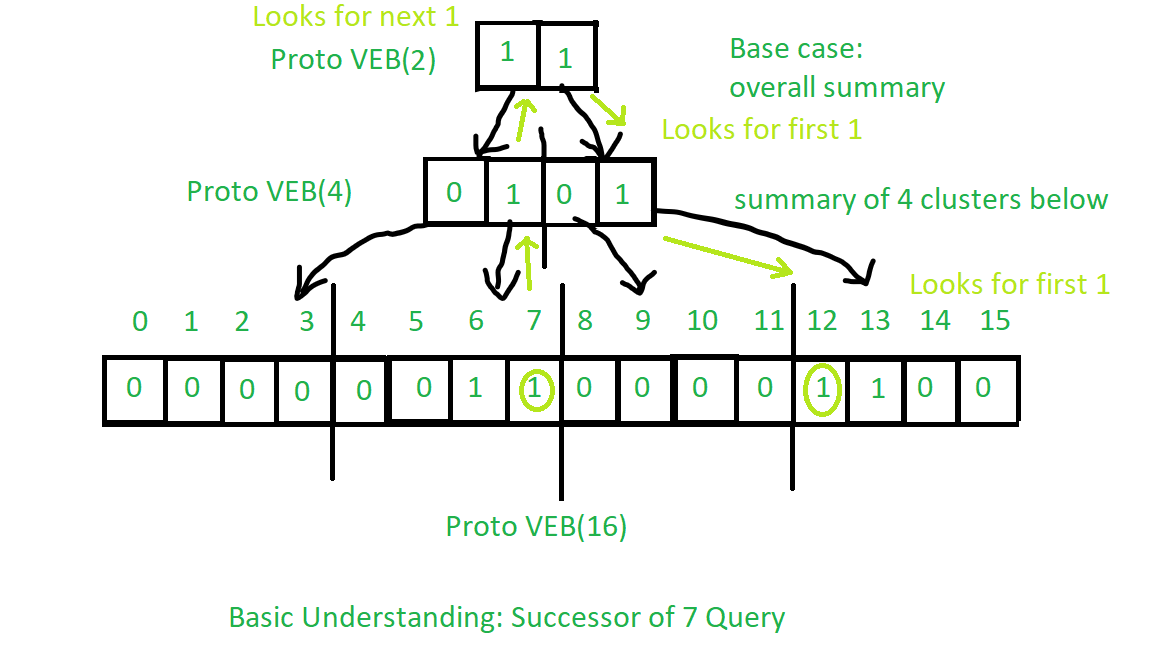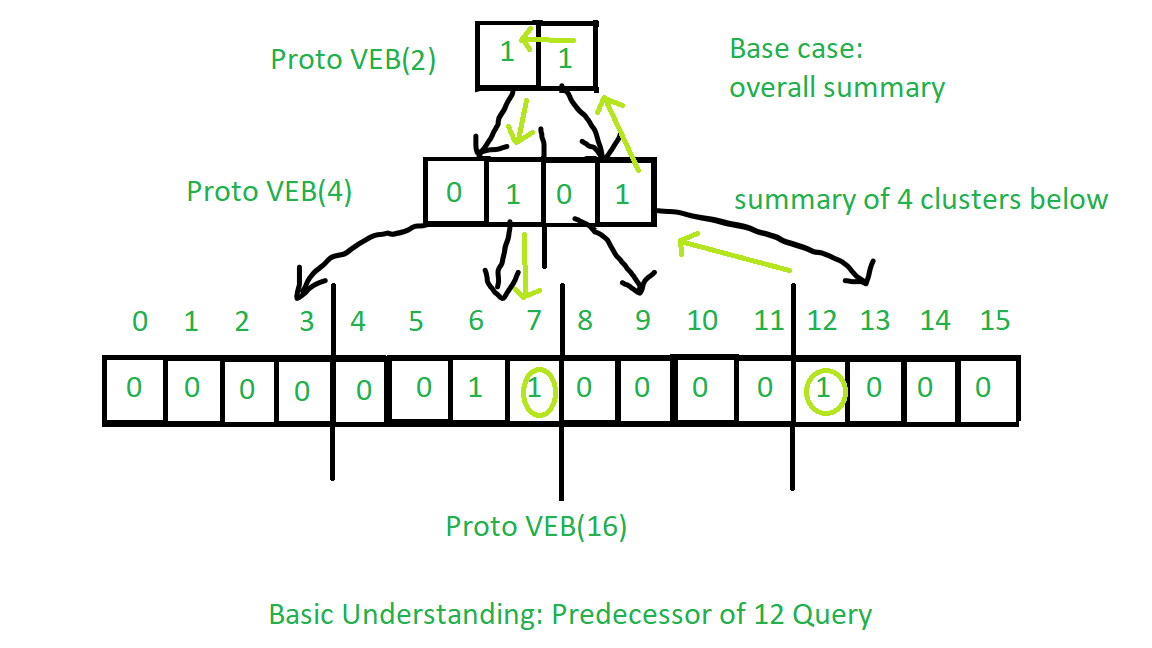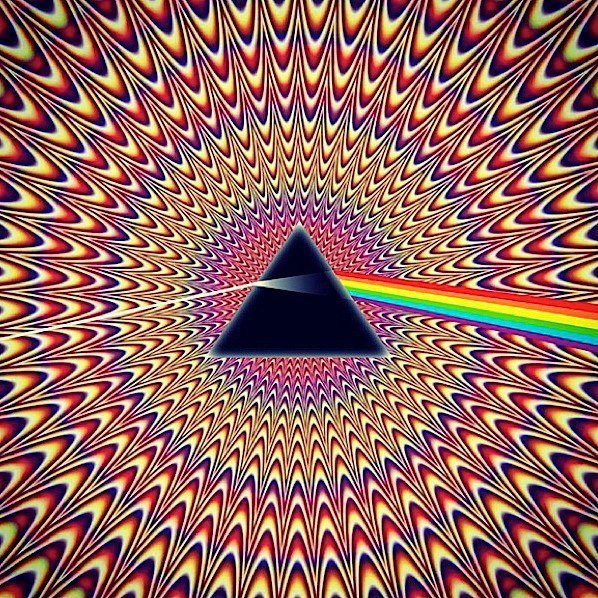# Proto Van Emde Boas Tree | Set 6 | Query : Successor and Predecessor

Please refer all previous articles on Proto Van Emde Boas Tree first.

Successor Query Procedure:

1. Base case: For Proto-VEB of size 2 the only possibility is that key is 0 and if the next key is present then it is its successor or there is no successor. So the same procedure is applied.
2. Recursion:
• First, we will look in the present cluster (means the cluster in which the query key is present) if there is any key greater than query key is present then we will be the successor so we return it.
• If above is not the case then we will recursively call successor procedure over summary to find next true value in summary. If there is no next true value in summary then we will return -1 as a sign that no larger key is present.
• In the above operation if we find any next true value then we will find the minimum key present in that cluster which will be the successor of the query key.

See the image below for basic understanding of the operation of Successor query:Procedure for Predecessor is same as successor with some minor changes you should try to understand it from the above description for successor query. See the image below for basic understanding:Below is the implementation:

 // C++ implementation of the approach  #include  using namespace std;     class Proto_Van_Emde_Boas {  public:      // Total number of keys      int universe_size;         // Summary      Proto_Van_Emde_Boas* summary;         // Clusters array of Proto-VEB pointers      vector clusters;         int root(int u)      {          return int(sqrt(u));      }         // Function to return cluster numbers      // in which key is present      int high(int x)      {          return x / root(universe_size);      }         // Function to return position of x in cluster      int low(int x)      {          return x % root(universe_size);      }         // Function to return the index from      // cluster number and position      int generate_index(int cluster, int position)      {          return cluster * root(universe_size) + position;      }         // Constructor      Proto_Van_Emde_Boas(int size)      {          universe_size = size;             // Base case          if (size <= 2) {                 // Set summary to nullptr as there is no              // more summary for size 2              summary = nullptr;                 // Vector of two pointers              // nullptr in starting              clusters = vector(size, nullptr);          }          else {                 // Assiging Proto-VEB(sqrt(u)) to summary              summary = new Proto_Van_Emde_Boas(root(size));                 // Creating array of Proto-VEB Tree pointers of size sqrt(u)              // first all nullptrs are going to assign              clusters = vector(root(size), nullptr);                 // Assigning Proto-VEB(sqrt(u)) to all its clusters              for (int i = 0; i < root(size); i++) {                  clusters[i] = new Proto_Van_Emde_Boas(root(size));              }          }      }  };     // Function that returns true if the  // key is present in the tree  bool isMember(Proto_Van_Emde_Boas* helper, int key)  {         // If key is greater then universe_size then      // returns false      if (key >= helper->universe_size)          return false;         // If we reach at base case      // the just return whether      // pointer is nullptr then false      // else return true      if (helper->universe_size == 2) {          return helper->clusters[key];      }      else {             // Recursively go deep into the          // level of Proto-VEB tree using its          // cluster index and its position          return isMember(helper->clusters[helper->high(key)],                          helper->low(key));      }  }     // Function to insert a key in the tree  void insert(Proto_Van_Emde_Boas*& helper, int key)  {      // If we reach at base case      // then assign Proto-VEB(1) in place      // of nullptr      if (helper->universe_size == 2) {          helper->clusters[key] = new Proto_Van_Emde_Boas(1);      }      else {             // Recursively using index of cluster and its          // position in cluster          insert(helper->clusters[helper->high(key)],                 helper->low(key));             // Also do the same recusion in summary VEB          insert(helper->summary, helper->high(key));      }  }     // Function to return the minimum key from the tree  int minimum(Proto_Van_Emde_Boas* helper)  {      // Base case chooses the least key      // present in the cluster      if (helper->universe_size == 2) {          if (helper->clusters) {              return 0;          }          else if (helper->clusters) {              return 1;          }             // No keys present then return -1          return -1;      }      else {             // Recursively find in summary for          // first 1 present in Proto-VEB          int minimum_cluster = minimum(helper->summary);          int offset;             // If no key is present in          // the cluster then return -1          if (minimum_cluster == -1) {              return -1;          }          else {                 // Recursively find the position of the key              // in the minimum_cluster              offset = minimum(helper->clusters[minimum_cluster]);                 // Returns overall index of minimum key              return helper->generate_index(minimum_cluster, offset);          }      }  }     // Function to return the maximum key from the tree  int maximum(Proto_Van_Emde_Boas* helper)  {         // Return the maximum key present in      // the cluster      if (helper->universe_size == 2) {          if (helper->clusters) {              return 1;          }          else if (helper->clusters) {              return 0;          }             // Return -1 if no keys present in the          // cluster          return -1;      }      else {             // Recursively find the last 1 present          // in the summary          int maximum_cluster = maximum(helper->summary);          int offset;             // If no key is present in          // the cluster then return -1          if (maximum_cluster == -1) {              return -1;          }          else {                 // Recursively find the position of the key              // in the maximum_cluster              offset = maximum(helper->clusters[maximum_cluster]);              return helper->generate_index(maximum_cluster, offset);          }      }  }     // Function to return the successor of key in the tree  int successor(Proto_Van_Emde_Boas* helper, int key)  {      // Base case, returns key greater than      // our query key in the cluster if present      // else returns -1      if (helper->universe_size == 2) {          if (key == 0 && helper->clusters)              return 1;          else             return -1;      }      else {             // Check if any key is greater than query key in the cluster          int offset = successor(helper->clusters[helper->high(key)],                                 helper->low(key));             // If it is present then return its index          if (offset != -1)              return helper->generate_index(helper->high(key), offset);          else {                 // If no successor is present within the cluster then              // go to the summmary and find the next summary with              // key present(1) named successor_cluster              int successor_cluster = successor(helper->summary,                                                helper->high(key));                 // If no next 1 in the summary then return -1              if (successor_cluster == -1)                  return -1;              else {                     // Find the minimum key in the successor_cluster                  offset = minimum(helper->clusters[successor_cluster]);                     // Generate its index and return                  return helper->generate_index(successor_cluster, offset);              }          }      }  }     // Function to return the predecessor of key in the tree  int predecessor(Proto_Van_Emde_Boas* helper, int key)  {         // Base case, find smaller key present in      // the cluster      // If present else return -1      if (helper->universe_size == 2) {          if (key == 1 && helper->clusters)              return 0;          else             return -1;      }      else {             // Check if any key is lower than query key in the cluster          int offset = predecessor(helper->clusters[helper->high(key)],                                   helper->low(key));             // If it is present then return its index          if (offset != -1)              return helper->generate_index(helper->high(key), offset);          else {                 // If no predecessor is present within the cluster then              // go to the summmary and find the next summary with              // key present(1) named predecessor_cluster              int predecessor_cluster = predecessor(helper->summary,                                                    helper->high(key));                 // If no next 1 in the summary then return -1              if (predecessor_cluster == -1)                  return -1;              else {                     // Find the maximum key in the predecessor_cluster                  offset = maximum(helper->clusters[predecessor_cluster]);                     // Generate its index and return                  return helper->generate_index(predecessor_cluster, offset);              }          }      }  }     // Function to delete a key from the tree  void pveb_delete(Proto_Van_Emde_Boas*& helper, int key)  {         // Base case: If the key is present      // then make it nullptr      if (helper->universe_size == 2) {          if (helper->clusters[key]) {              delete helper->clusters[key];              helper->clusters[key] = nullptr;          }      }      else {             // Recursive delete to reach at the base case          pveb_delete(helper->clusters[helper->high(key)], helper->low(key));             bool isanyinCluster = false;             // Iterate over the cluster of keys to check whether          // any other key is present within that cluster          // If yes then we should not update summary to 0          // else update summary to 0          for (int i = helper->high(key) * helper->root(helper->universe_size);               i < (helper->high(key) + 1) * helper->root(helper->universe_size);               i++) {                 // If member is present then break the loop              if (isMember(helper->clusters[helper->high(key)], i)) {                  isanyinCluster = true;                  break;              }          }             // If no member is present then          // update summary to zero          if (isanyinCluster == false) {              pveb_delete(helper->summary, helper->high(key));          }      }  }     // Driver code  int main()  {      Proto_Van_Emde_Boas* hello = new Proto_Van_Emde_Boas(16);         cout << boolalpha;         insert(hello, 2);         insert(hello, 13);         insert(hello, 3);         cout << successor(hello, 3) << endl;         cout << predecessor(hello, 13) << endl;  }

Recurrence Relation for Successor and Predecessor Queries:

T(u) = T(u) = 2T()) + O(log2())

Time Complexity : O(log2(u)*log2(log2(u)))

Attention reader! Don’t stop learning now. Get hold of all the important DSA concepts with the DSA Self Paced Course at a student-friendly price and become industry ready.

My Personal Notes arrow_drop_upCheck out this Author's contributed articles.

If you like GeeksforGeeks and would like to contribute, you can also write an article using contribute.geeksforgeeks.org or mail your article to contribute@geeksforgeeks.org. See your article appearing on the GeeksforGeeks main page and help other Geeks.

Please Improve this article if you find anything incorrect by clicking on the "Improve Article" button below.